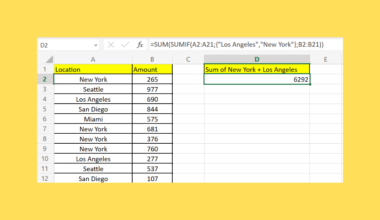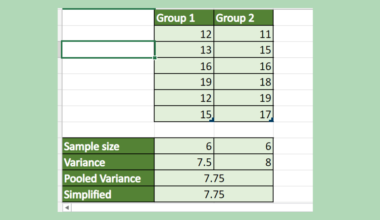# How to Calculate Cosine Similarity in Excel

This is the ultimate guide on how to calculate cosine similarity in Excel.

Excel is a powerful tool that is popularly used for calculations. Since it contains multiple built-in functions and features that we can combine to create formulas, we can perform almost all sorts of calculations in Excel.

And one of these is cosine similarity. So cosine similarity is a type of metric that is used to measure the similarity of two vectors. Essentially, cosine similarity measures the similarity in the orientation or direction of two vectors.

But, cosine similarity ignores the differences in the magnitude or scale of the vectors. Furthermore, the two vectors need to be part of the same inner product space, meaning that both vectors must produce a scalar through inner product multiplication.

So the similarity of the two vectors is obtained by the cosine of the angle between them. Mathematically, cosine similarity is defined as the dot product of the two vectors divided by the magnitude of the two vectors.

Moreover, we will combine built-in functions to create a formula to calculate cosine similarity in Excel.

Let’s take a sample scenario wherein we need to calculate the cosine similarity in Excel.

Suppose you have two data sets containing a list of values. And you are tasked to calculate the cosine similarity between the two vectors or data sets. To make your work easier, you opted to perform it in Excel by combining different built-in functions to create a formula.

Before we move on to a real example, let’s first explain how to write some functions we will use to calculate cosine similarity in Excel.

## The Anatomy of the SUMPRODUCT Function

The syntax or the way we write the `SUMPRODUCT` function is as follows:

`=SUMPRODUCT(array1, [array2], [array3],...)`

Let’s take apart this formula and understand what each term means:

• = the equal sign is how we activate any function in Excel.
• SUMPRODUCT() refers to our `SUMPRODUCT` function. And this function is used to return the sum of the products of the selected ranges or arrays.
• array1 is a required argument. So this refers to 2 to 255 arrays that we want to multiply and then add the components. Additionally, all the arrays must have the same dimensions.
• array2 is an optional argument. And this is also referring to 2 to 255 arrays which we will select to multiply and add the components. Furthermore, we usually input the cell reference of the range containing the array.

## The Anatomy of the SUMSQ Function

The syntax or the way we write the `SUMSQ` function is as follows:

`=SUMSQ(number1, [number2],...)`

Let’s take apart this formula and understand what each term means:

• = the equal sign is how we begin any function in Excel.
• SUMSQ() refers to our `SUMSQ` function. And this function is used to return the sum of the squares of the inputted arguments. So the arguments can be numbers, arrays, names, or cell references containing the needed values or numbers.
• number1 is a required argument. So this can be 1 to 255 numbers, arrays, names, or cell references containing the numbers we want to use to calculate the sum of the squares.
• number2 is an optional argument. And this is also referring to 1 to 255 numbers, arrays, names, or cell references containing the needed numbers which we want to use to get the sum of the squares.

Great! Now we can dive into a real example of calculating cosine similarity in Excel.

## A Real Example of Calculating Cosine Similarity in Excel

Let’s say we have two data sets containing a list of values. So we have data set A and data set B, which have the same number of values. In this case, our initial data set would look like this:Since cosine similarity is a measure of the similarity between two vectors having the same inner product space, the two data sets represent our two vectors.

Additionally, cosine similarity is calculated using the formula `= ΣAiBi / (√ΣAi2√ΣBi2)`. So we will get the cosine angle between the two vectors to get the cosine similarity.

Furthermore, we need to get the dot product between the two vectors. Then, we will divide the dot product by the magnitude of the vector. To perform this in Excel, we will use a formula combining the `SUMPRODUCT` function, the `SQRT` function, and the `SUMSQ` function.

So the `SUMPRODUCT` function is used to return the sum of the products. And the `SQRT` function is used to return the square root of a selected number. Then, the `SUMSQ` is used to get the sum of the squares of the inputted numbers or arrays.

When we combine these functions, we can create a formula to calculate the cosine similarity of the two vectors. Once we get the result, we can then interpret the cosine similarity value.

Firstly, a cosine similarity value of -1 indicates the maximum dissimilarity meaning the two vectors have little to no similarity. Secondly, a cosine similarity of 0 can indicate that the two vectors are orthogonal, which means the angle is perpendicular to each other.

Thirdly, a cosine similarity of 1 can indicate the maximum similarity meaning the two vectors are very similar or almost the same.

So our final data set would look like this:Amazing! Now we can move on and discuss the steps on how to calculate cosine similarity in Excel.

## How to Calculate Cosine Similarity in Excel

In this section, we will explain the step-by-step process of how to calculate cosine similarity in Excel. To apply this method to your work, we can simply follow the steps below.

1. Firstly, we must label our data sets to differentiate the two vectors. Then, we can simply proceed to use the formula to get the cosine similarity of our two vectors. So type in the formula “=SUMPRODUCT(B\$2:B\$7,C2:C7)/(SQRT(SUMSQ(C2:C7))*SQRT(SUMSQ(\$B\$2:\$B\$7)))”.

Lastly, we will press the Enter key to return the value. Additionally, we can try interpreting our cosine similarity value. Since we got a value of 0.931098676, this indicates very high similarity between the two data sets.2. And tada! We have successfully calculated cosine similarity in Excel.And that’s pretty much it! We have successfully discussed how to calculate cosine similarity in Excel. Now you can apply this learning to your work whenever you need to find the similarity between two vectors or data sets.

Are you interested in learning more about what Excel can do? You can now use the `SUMSQ` function and the various other Microsoft Excel formulas available to create great worksheets that work for you. Make sure to subscribe to our newsletter to be the first to know about the latest guides and tutorials from us.Our goal this year is to create lots of rich, bite-sized tutorials for Google Sheets users like you. If you liked this one, you'll love what we are working on! Readers receive ✨ early access ✨ to new content.

##### You May Also Like## How to Use SUMIF with OR in Excel

This guide will explain how to use SUMIF with OR logic in Excel. We can use the SUMIF…## How to Make an ANOVA Table in Excel

This guide will explain how to make an ANOVA table in Excel. ANOVA tables allow users to test…## How to Use SUMIFS on Multiple Columns with Several Criteria in Excel

This guide will explain how to find the sum of multiple columns given one or more criteria. We’ll…## How to Calculate a Sigmoid Function in Excel

This guide will explain how to calculate a sigmoid function in Excel. The sigmoid function’s S-shaped curve is…